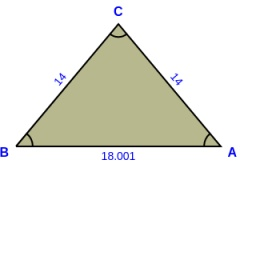# Calculate: 6686

We know the right angle γ, side b = 14 cm, and height vc = 8.8 cm in the right triangle ABC. Calculate:
angle α =
angle β =
page a =
page c =

A =  38.9448 °
B =  51.0552 °
a =  11.3147 cm
c =  18.0006 cm

### Step-by-step explanation:

Try calculation via our triangle calculator.Did you find an error or inaccuracy? Feel free to write us. Thank you!

Tips for related online calculators
The Pythagorean theorem is the base for the right triangle calculator.